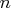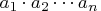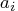Previous Page | Next Page

 Language Reference

## GEOMEAN Function

calculates geometric means

GEOMEAN( matrix)

where matrix is a numeric matrix of nonnegative values.

The GEOMEAN function returns a scalar containing the geometric mean of the elements of the input matrix. The geometric mean of a set of nonnegative numbersis theth root of the product.

The geometric mean is zero if any of theare zero. The geometric mean is not defined for negative inputs. If any of theare missing, they are excluded from the computation.

The geometric mean can be used to compute the average return on an investment. For example, the following data gives the annual returns on U.S. Treasury bonds from 1994 - 2004. The following statements compute that the average rate of return during this time was 6.43%:


/*         year  return% */
TBonds = { 1994  -8.04,
1995  23.48,
1996   1.43,
1997   9.94,
1998  14.92,
1999  -8.25,
2000  16.66,
2001   5.57,
2002  15.12,
2003   0.38,
2004   4.49 };

proportion = 1 + TBonds[,2]/100; /* convert to proportion */
aveReturn = geomean( proportion );
print aveReturn;

aveReturn

1.0643334Previous Page | Next Page | Top of Page